# Cosmic Blunder? Einstein’s Special Relativity and the Light-like Separated Events Equation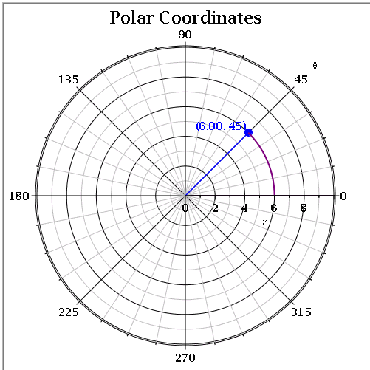The Assumptions that were listed in my last blog form the basis for what is known as ‘Einstein’s Special Relativity’. It is named with the term ‘Special’ because it applies to the case where the observers and the events in the frame are at a constant velocity (I.E. there is no acceleration being applied to anything anywhere in the system).

To summarize the postulates again we post the following.

The laws of physics are invariant, or identical in all inertial systems in non-accelerating frames of reference.

The speed of light in a vacuum is the same for all observers, regardless of the motion of the light source.

These rules were then formulated into a set of equations to describe them in mathematical terms in what is known as the Lorentz Transformations. The start of which is the following assumption for light-like separated events.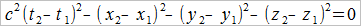Where did they get this equation?

When you stop being intimidated by the number of variables in this equation and realize that there’s a basic form to it, you can see where they derived it from. ‘Light-like separated events’ is a fancy term for the event where a light-ray travels from one point to another. Reverse engineer this equation and you’ll find yourself with a 3-dimensional version of a basic geometry problem. Let’s recall, in basic geometry: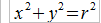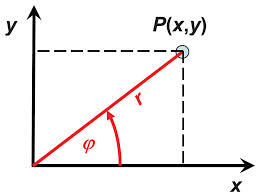The variable r is a radius formed by the Pythagorean Theorem, and the above equation is just one of two parts used to convert Cartesian Coordinates to a Radial Coordinate System. The ‘Light-like separated events’ equation is just a rearrangement of a 3-dimensional version of the Pythagorean Theorem, which is as follows:

Whereis the 3-Dimensional radius of our 3-Dimensional Cartesian-Coordinates. To get the ‘Light-like separated events’ equation back into its original form, we have to observe a couple things about it.

1. c is a speed. It is a translation in space covered over a period of time.
2.are all merely translations. They are not velocity vector components, but merely the distance covered in a certain direction with no time component.

These two observations are important because we can now detect the time-component that is hidden from us by the term. Let us rearrange the ‘Light-like separated events’ equation so that the translation components are separated from the speed components.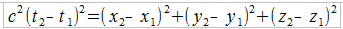That looks a little more familiar, now, doesn’t it? Let’s make one more observation. That is: a distance covered between two points of time is a speed, or velocity. Also, a speed component that is multiplied by a span of time becomes a distance covered, or a translation. There are two ways that we can now reach the original form of the equation that they derived our ‘Light-like separated events’. If is a speed, then it is a distance covered divided by a difference in time.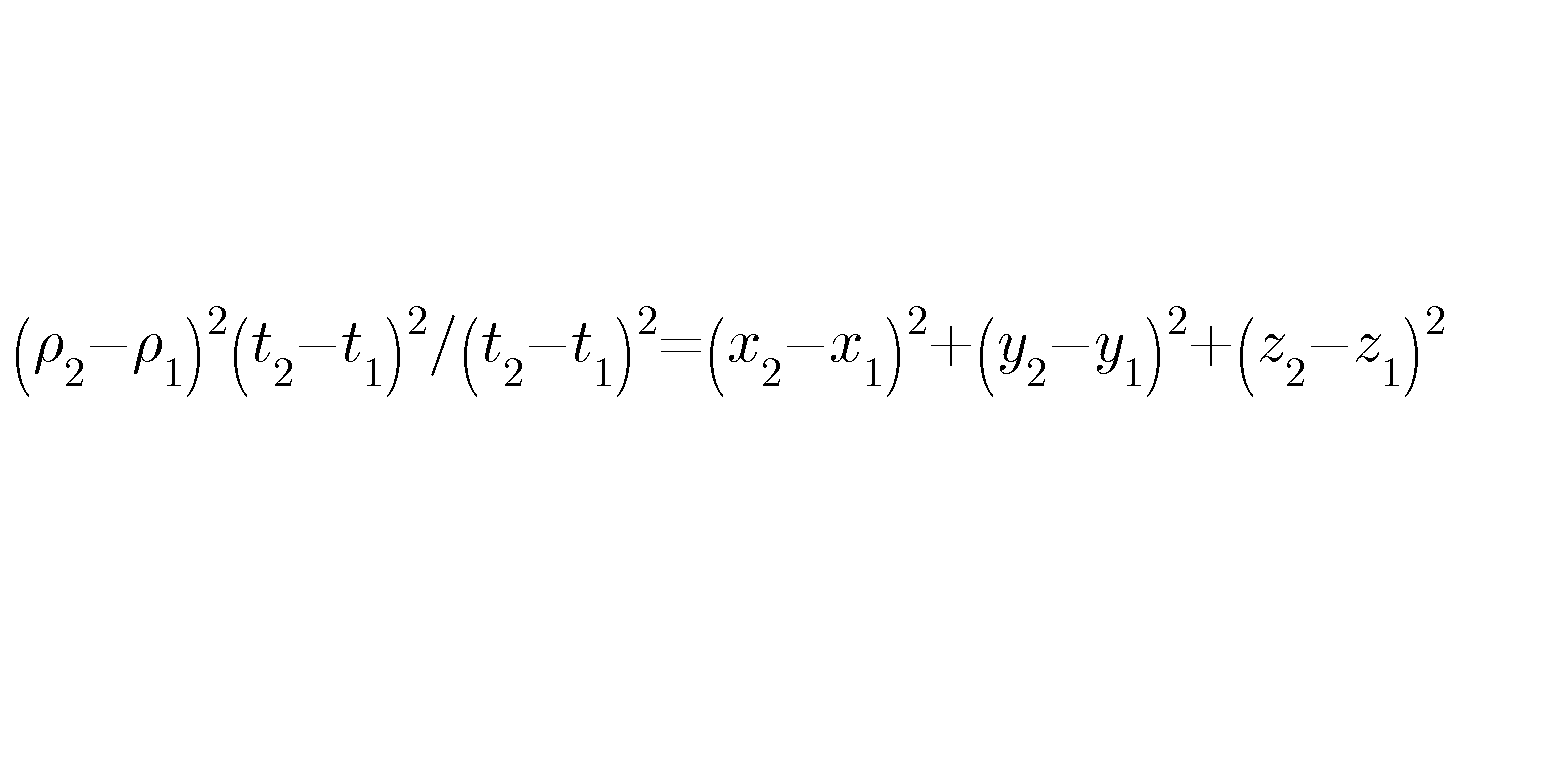Did you see what we did there? By separating the speed of light into its distance and time components, we now have a piece of the denominator and numerator that cancel each other out. So we get our Pythagorean Theorem in the form of distance covered:Alternatively, we can divide the original equation by the time components to turn the Cartesian translation into speed components.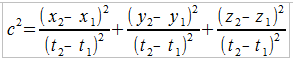You can now express the right-hand side in the speed components of a Velocity vector to make it more readable.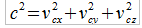That’s all this is. The beginning of Lorenz’s transformation can be easily concluded to be deeply flawed in its logic from the very beginning. Based on what we know from above we can observe the following.

In the concept of space-time, time is treated as a fourth dimension amongst its Cartesian coordinates. The “light-like separated events” equation does this by displaying time multiplied by the speed of light as a way of demonstrating it as a fourth coordinate. But the “light-like separated events equation suffers from the fact that the speed of light is merely a distance covered divided by the same time components that it is multiplied by in the original equation. A speed multiplied by a time-span merely becomes a distance covered with no speed components whatsoever, so you cannot treat time as a fourth dimension, because that dimension immediately cancels itself out in this equation.